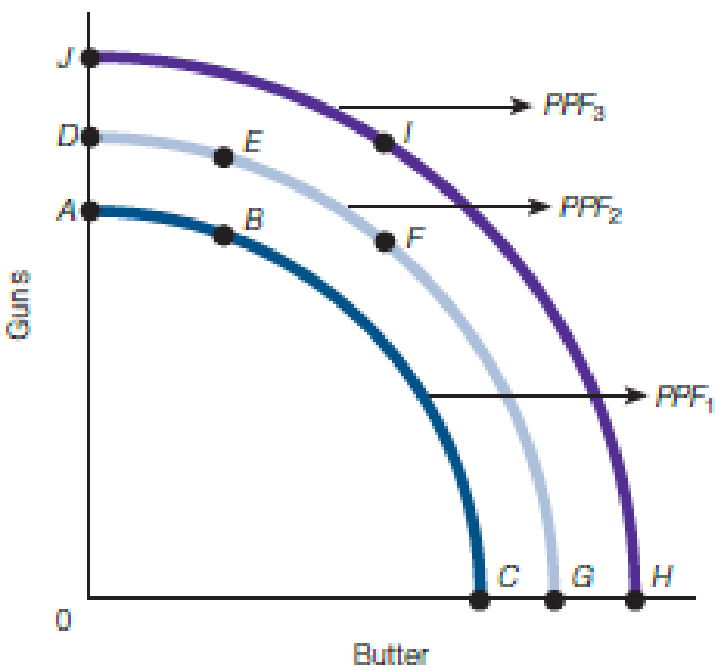Chapter 2, Problem 6WNG

Chapter
Section
Textbook Problem

If PPF2 in the graph that follows is the relevant PPF, then which points are unattainable? Explain your answer.To determine

Explain which point is unattainable.

Explanation

Figure 1 shows the different PPF of guns and butter below:

In figure 1, the vertical axis measures the quantity of guns and horizontal axis measures the quantity of butter. PPF1, PPF2, and PPF3 are different production possibility frontier...

Still sussing out bartleby?

Check out a sample textbook solution.

See a sample solution

The Solution to Your Study Problems

Bartleby provides explanations to thousands of textbook problems written by our experts, many with advanced degrees!

Get Started

Find more solutions based on key concepts